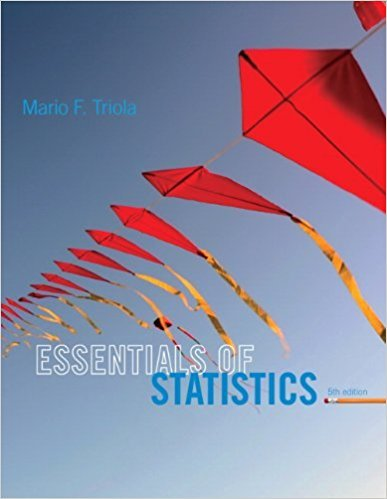×
×

# Solutions for Chapter 9.4: Two Dependent Samples (Matched Pairs)## Full solutions for Essentials of Statistics | 5th Edition

ISBN: 9780321924599Solutions for Chapter 9.4: Two Dependent Samples (Matched Pairs)

Solutions for Chapter 9.4
4 5 0 344 Reviews
28
4
##### ISBN: 9780321924599

Essentials of Statistics was written by and is associated to the ISBN: 9780321924599. Since 25 problems in chapter 9.4: Two Dependent Samples (Matched Pairs) have been answered, more than 15673 students have viewed full step-by-step solutions from this chapter. This expansive textbook survival guide covers the following chapters and their solutions. Chapter 9.4: Two Dependent Samples (Matched Pairs) includes 25 full step-by-step solutions. This textbook survival guide was created for the textbook: Essentials of Statistics, edition: 5.

Key Statistics Terms and definitions covered in this textbook
• Alias

In a fractional factorial experiment when certain factor effects cannot be estimated uniquely, they are said to be aliased.

• All possible (subsets) regressions

A method of variable selection in regression that examines all possible subsets of the candidate regressor variables. Eficient computer algorithms have been developed for implementing all possible regressions

• Analysis of variance (ANOVA)

A method of decomposing the total variability in a set of observations, as measured by the sum of the squares of these observations from their average, into component sums of squares that are associated with speciic deined sources of variation

• Analytic study

A study in which a sample from a population is used to make inference to a future population. Stability needs to be assumed. See Enumerative study

• Axioms of probability

A set of rules that probabilities deined on a sample space must follow. See Probability

• Bayes’ theorem

An equation for a conditional probability such as PA B ( | ) in terms of the reverse conditional probability PB A ( | ).

• Box plot (or box and whisker plot)

A graphical display of data in which the box contains the middle 50% of the data (the interquartile range) with the median dividing it, and the whiskers extend to the smallest and largest values (or some deined lower and upper limits).

• Chance cause

The portion of the variability in a set of observations that is due to only random forces and which cannot be traced to speciic sources, such as operators, materials, or equipment. Also called a common cause.

• Components of variance

The individual components of the total variance that are attributable to speciic sources. This usually refers to the individual variance components arising from a random or mixed model analysis of variance.

• Conidence level

Another term for the conidence coeficient.

• Control chart

A graphical display used to monitor a process. It usually consists of a horizontal center line corresponding to the in-control value of the parameter that is being monitored and lower and upper control limits. The control limits are determined by statistical criteria and are not arbitrary, nor are they related to speciication limits. If sample points fall within the control limits, the process is said to be in-control, or free from assignable causes. Points beyond the control limits indicate an out-of-control process; that is, assignable causes are likely present. This signals the need to ind and remove the assignable causes.

• Deming’s 14 points.

A management philosophy promoted by W. Edwards Deming that emphasizes the importance of change and quality

• Designed experiment

An experiment in which the tests are planned in advance and the plans usually incorporate statistical models. See Experiment

• Discrete distribution

A probability distribution for a discrete random variable

• Discrete uniform random variable

A discrete random variable with a inite range and constant probability mass function.

• Dispersion

The amount of variability exhibited by data

• Empirical model

A model to relate a response to one or more regressors or factors that is developed from data obtained from the system.

• Estimate (or point estimate)

The numerical value of a point estimator.

• Gamma random variable

A random variable that generalizes an Erlang random variable to noninteger values of the parameter r

• Gaussian distribution

Another name for the normal distribution, based on the strong connection of Karl F. Gauss to the normal distribution; often used in physics and electrical engineering applications

×